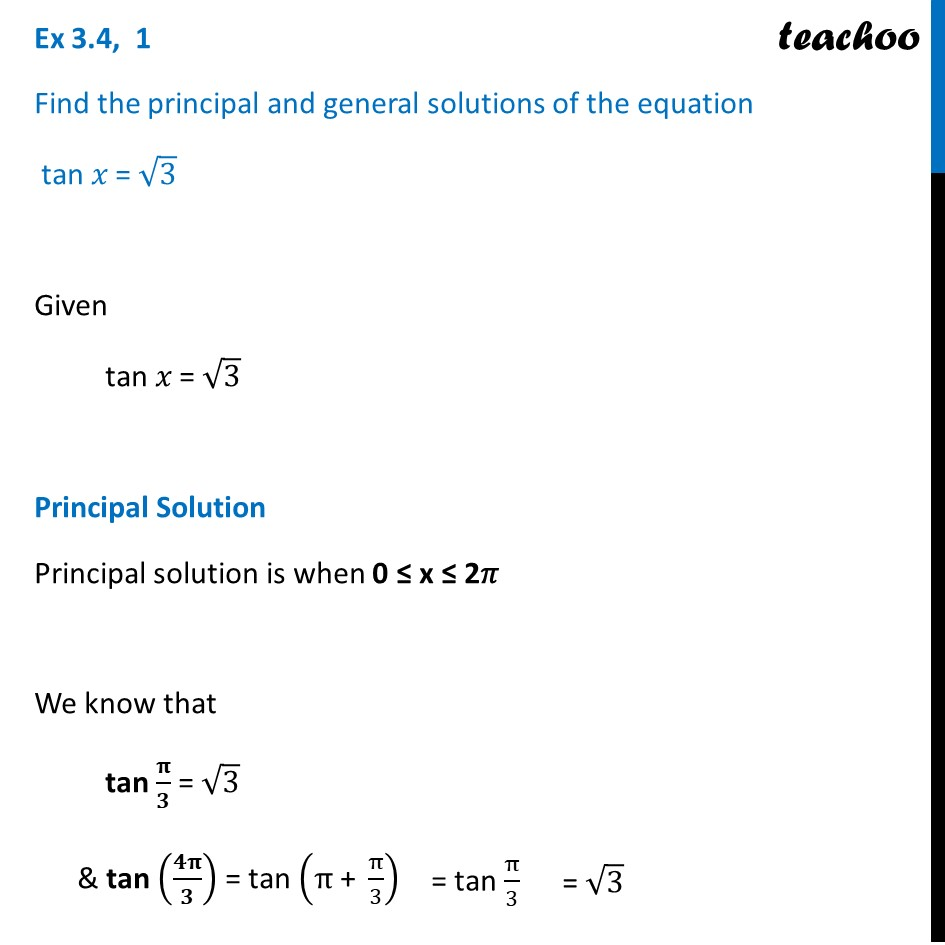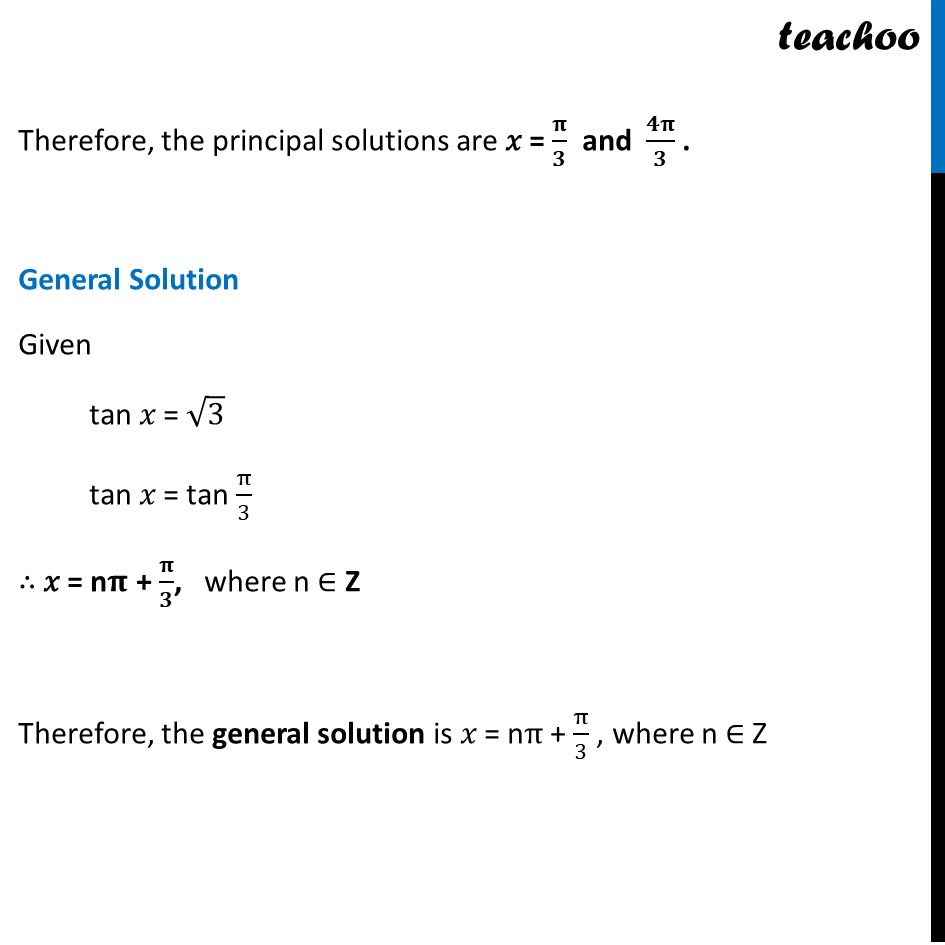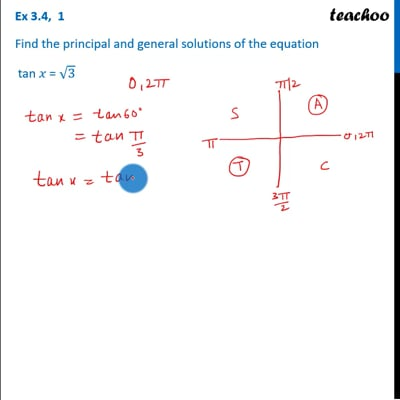Finding General Solutions

Chapter 3 Class 11 Trigonometric Functions
Concept wiseThis video is only available for Teachoo black users

Introducing your new favourite teacher - Teachoo Black, at only ₹83 per month

### Transcript

Ex 3.4, 1 Find the principal and general solutions of the equation tan 𝑥 = √3 Given tan 𝑥 = √3 Principal Solution Principal solution is when 0 ≤ x ≤ 2𝜋 We know that tan 𝛑/𝟑 = √3 & tan (𝟒𝛑/𝟑) = tan (π" + " π/3) Therefore, the principal solutions are 𝑥 = 𝛑/𝟑 and 𝟒𝛑/𝟑 . General Solution Given tan 𝑥 = √3 tan 𝑥 = tan π/3 ∴ 𝑥 = n𝛑 + 𝛑/𝟑, where n ∈ Z Therefore, the general solution is 𝑥 = nπ + π/3 , where n ∈ Z